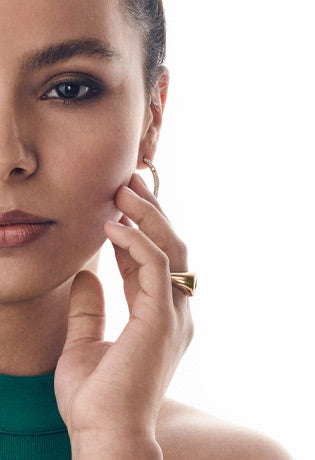## OUR IMPACT

We're not just creating diamonds, we're crafting a better tomorrow. Our gems are more than just symbols of love and commitment – they are active participants in the healing of our planet. To become a caretaker of the future is to embrace environmental stewardship through all facets of life.

## · T H E F U T U R E I S A L U X U R Y · T H E F U T U R E I S A L U X U R Y · T H E F U T U R E I S A L U X U R Y · T H E F U T U R E I S A L U X U R Y · T H E F U T U R E I S A L U X U R Y · T H E F U T U R E I S A L U X U R Y · T H E F U T U R E I S A L U X U R Y · T H E F U T U R E I S A L U X U R Y · T H E F U T U R E I S A L U X U R Y · T H E F U T U R E I S A L U X U R Y · T H E F U T U R E I S A L U X U R Y · T H E F U T U R E I S A L U X U R Y · T H E F U T U R E I S A L U X U R Y · T H E F U T U R E I S A L U X U R Y · T H E F U T U R E I S A L U X U R Y · T H E F U T U R E I S A L U X U R Y · T H E F U T U R E I S A L U X U R Y · T H E F U T U R E I S A L U X U R Y · T H E F U T U R E I S A L U X U R Y · T H E F U T U R E I S A L U X U R Y · T H E F U T U R E I S A L U X U R Y · T H E F U T U R E I S A L U X U R Y · T H E F U T U R E I S A L U X U R Y · T H E F U T U R E I S A L U X U R Y · T H E F U T U R E I S A L U X U R Y